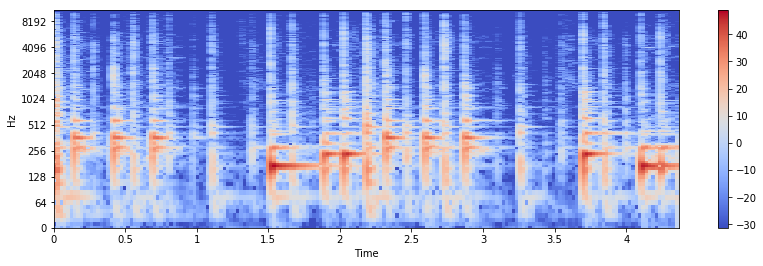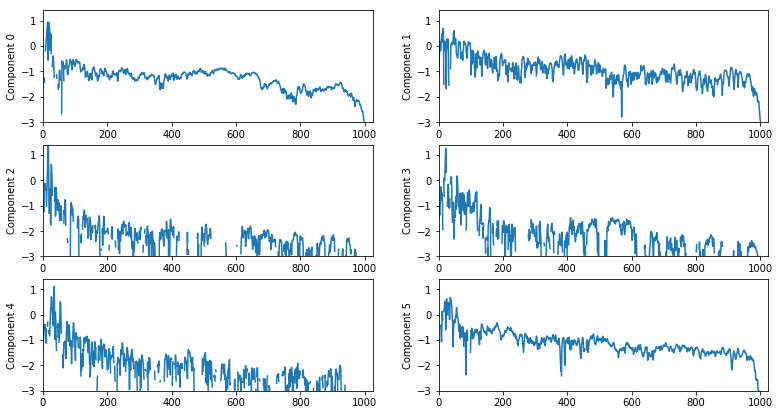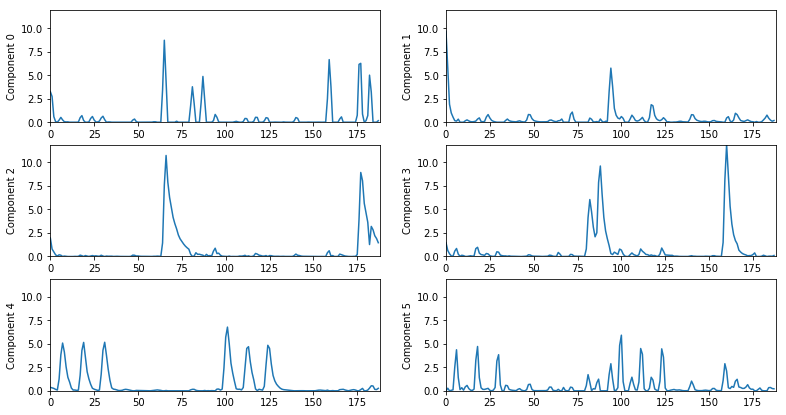# Nonnegative Matrix Factorization¶

Nonnegative matrix factorization (NMF) is an algorithm that factorizes a nonnegative matrix, $X$, into a product of two nonnegative matrices, $W$ and $H$. It is an unsupervised iterative algorithm that minimizes a distance between $X$ and the product $WH$:

$$\min_{W, H} d(X, WH)$$

If $X$ has dimensions $M$ by $N$, then $W$ will have dimensions $M$ by $R$, and $H$ will have dimensions $R$ by $N$, where inner dimension $R$ is the rank or number of components of the decomposition.

When applied to a musical signal, we find that NMF can decompose the signal into separate note events. Therefore, NMF is quite useful and popular for tasks such as transcription and source separation.

The input, $X$, is often a magnitude spectrogram. In such a case, we find that the columns of $W$ represent spectra of note events, and the rows of $H$ represent temporal envelopes of the same note events.

In :
x, sr = librosa.load('audio/conga_groove.wav')

Out:

Compute the STFT:

In :
S = librosa.stft(x)

Out:
<matplotlib.colorbar.Colorbar at 0x10c59e6d8>## librosa.decompose.decompose¶

We will use librosa.decompose.decompose to perform our factorization. librosa uses sklearn.decomposition.NMF by default as its factorization method.

In :
X, X_phase = librosa.magphase(S)
n_components = 6
W, H = librosa.decompose.decompose(X, n_components=n_components, sort=True)

In :
print(W.shape)
print(H.shape)

(1025, 6)
(6, 188)


Let's display the spectral profiles, $\{w_1, ..., w_R\}$:Let's display the temporal activations, $\{h_1, ..., h_R\}$:Finally, re-create the individual components, and listen to them. To do this, we will reconstruct the magnitude spectrogram from the NMF outputs and use the phase spectrogram from the original signal.

Component 0: